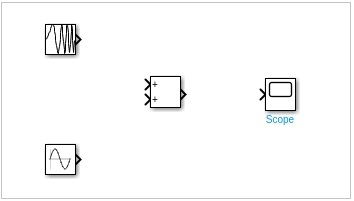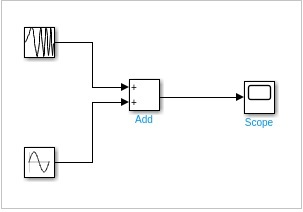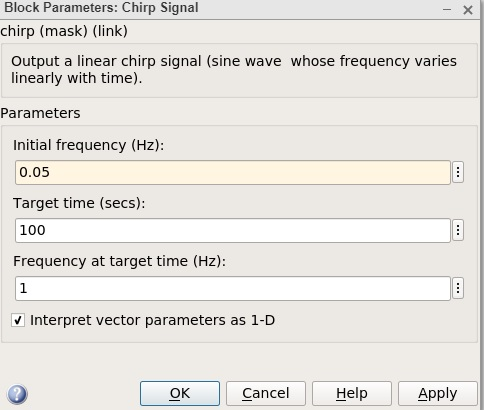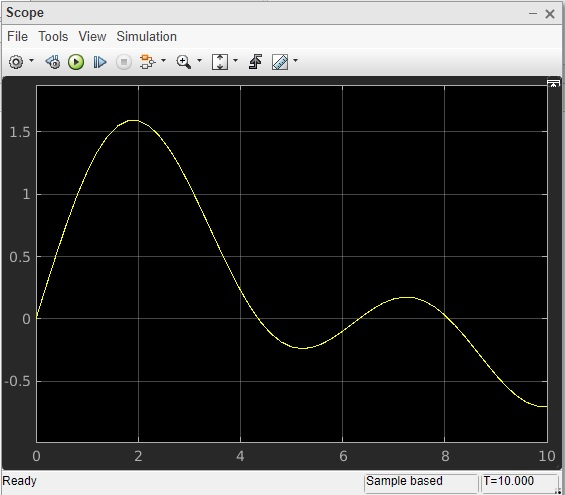# MATLAB Simulink - Mathematical Library

#### Data Preprocessing for Machine Learning using MATLAB

30 Lectures 4 hours

#### Image Processing Toolbox in MATLAB

17 Lectures 3 hours

#### Matlab - The Complete Course

Best Seller

37 Lectures 5 hours

In this chapter, we will learn how to sum the two given signals and get the output.

Select the blank model and open Simulink library browser as shown below −We are going to combine chirp signal and sine wave blocks by using add block from Mathoperation and see the final display.

Let us pick the block we want. Select chirp signal and sine wave from sources library, add block from math operations, scope block from sinks library.Join the lines to each block.Double click chirp signal and change the initial frequency from 0.1 to 0.05 and click on Ok button.The other blocks are kept as the default values. Now, click on run to see the output in scope as shown below.